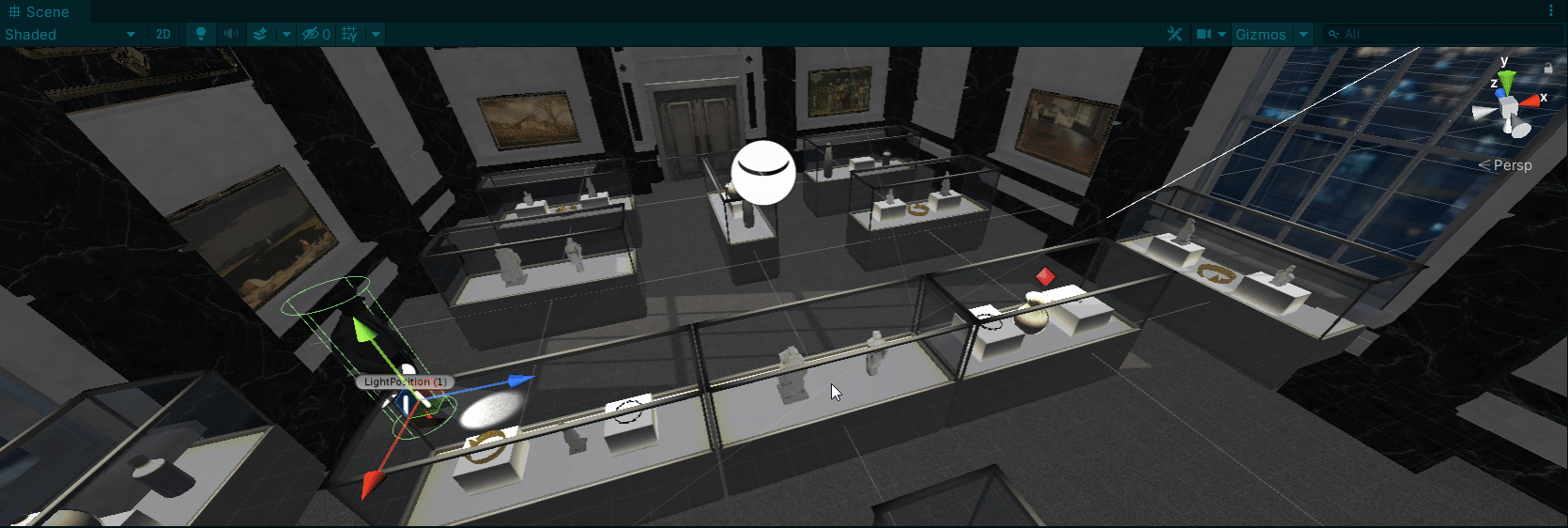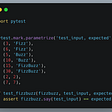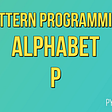# ZigZag Conversion — Day 71(Python)

Today’s question is based on Strings. This question is commonly asked among Paypal’s interviewers. This question is a medium tagged question Leetcode.

6. ZigZag Conversion

The string `"PAYPALISHIRING"` is written in a zigzag pattern on a given number of rows like this: (you may want to display this pattern in a fixed font for better legibility)

`P   A   H   NA P L S I I GY   I   R`

And then read line by line: `"PAHNAPLSIIGYIR"`

Write the code that will take a string and make this conversion given a number of rows:

`string convert(string s, int numRows);`

Example 1:

`Input: s = "PAYPALISHIRING", numRows = 3Output: "PAHNAPLSIIGYIR"`

Example 2:

`Input: s = "PAYPALISHIRING", numRows = 4Output: "PINALSIGYAHRPI"Explanation:P     I    NA   L S  I GY A   H RP     I`

Example 3:

`Input: s = "A", numRows = 1Output: "A"`

Constraints:

• `1 <= s.length <= 1000`
• `s` consists of English letters (lower-case and upper-case), `','` and `'.'`.
• `1 <= numRows <= 1000`

One way to solve this problem is to create a list of the required sizes and then fill each cell with the letters from the input string.

What would the dimensions of this list be? If we look at the example, we realize, the length of the list is already is given to us.

Let us take an example and solve,

We create a list of size 3

Next, we will place each letter in the string into this list until we reach the end of the list.

Once we reach the end of the list, we need to change the direction in which we append the characters in the list. Earlier, we were moving from Up to Down, now, we will reverse the direction from Down to Up.

We will again reverse the direction once we reach the 0th index in the list.

Continue until we have hit all the characters in the string.

In the end, we join all the elements in the list and return it.

To keep track of directions, we can have a flag that will tell us, when we should be moving from Up to Down or when to use a reverse direction.

Let us look into the code snippet.

`class Solution:    def convert(self, s: str, numRows: int) -> str:        if(numRows  < 2):            return s        arr = ['' for i in range(numRows)]        direction = 'down'        row = 0        for i in s:            arr[row] += i            if row == numRows-1:                direction = 'up'            elif row == 0:                direction = 'down'            if(direction == 'down'):                row += 1            else:                row -= 1        return(''.join(arr))`

One of the edge cases to remember is when the number of input rows is less than 2. In such cases, we return the string as it is.

Complexity analysis.

Time Complexity

We are visiting each character in the string. Hence the time complexity is O(N), where N is the number of characters.

Space Complexity.

We create a list of size the number of rows. Hence the space complexity is O(r), where r is the number of rows.

--

--

--

## More from Annamariya Tharayil

Software Engineer. Find me @ www.linkedin.com/in/annamariya-jt

Love podcasts or audiobooks? Learn on the go with our new app.

## Practical Inversion of Control in TypeScript with a Functional Twist## November 2018 Po.et Engineering Progress Report## How to Connect an Android Phone to a Docker Container ?## Doing the rounds- A quick guide to creating a modular waypoint system in unity## Getting to Know Pytest: A Simple and Effective Testing Tool## CS373 Spring 2022: Nathan Whyte## Spectrum Management in Autonomous Wireless Networks## Annamariya Tharayil

Software Engineer. Find me @ www.linkedin.com/in/annamariya-jt

## How to Print Alphabet P in Python?## Coding improves problem solving## Number of Good Pairs — Day 101(Python)## Python’s .sort() vs sorted()